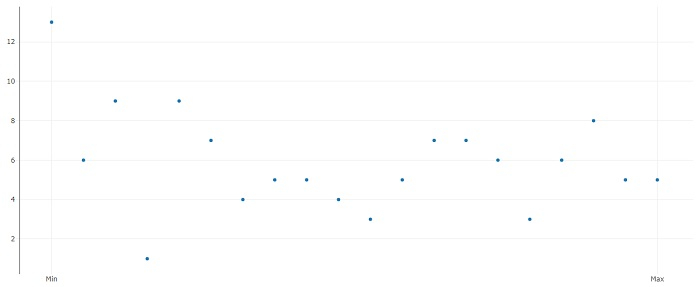How to display X-axis tick marks as minimum and maximum only without their values using plotly in R?

R ProgrammingServer Side ProgrammingProgramming

To display X-axis tick marks as minimum and maximum only without their values using plotly, we can use layout function of plot_ly package where we can pass the values for minimum and maximum using xaxis argument and the text using ticktext argument as shown in the below example.

Example

Following snippet creates a sample data frame −

x<-1:20
y<-rpois(20,5)
df<-data.frame(x,y)
df

output

The following dataframe is created −

x  y
1   1 13
2   2  6
3   3  9
4   4  1
5   5  9
6   6  7
7   7  4
8   8  5
9   9  5
10 10  4
11 11  3
12 12  5
13 13  7
14 14  7
15 15  6
16 16  3
17 17  6
18 18  8
19 19  5
20 20  5

To load plotly package and create point chart between x and y with minimum and maximum shown by Min and Max on X-axis, add the following code to the above snippet −

library(plotly)
plot_ly(data=df,x=x,y=y)%>%layout(xaxis=list(tickvals=c(1,20),ticktext=c("Min","Max")))

Output

If you execute all the above given snippets as a single program, it generates the following Output −